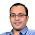### Array Problems - Contiguous Sum and Distinct Elements

Source: Posted by Algo Muse on contact page. Also posted on Algo Muse (December 2011) http://www.algomuse.appspot.com

Problem:

Two definitions:

1) Contiguous t-sum problem
Given an array A[1..n] and a number t as input, we want to find out if there exists a sub-array whose sum is t. For example, if the following array and t=8 is the input, the answer is YES since it contains the sub-array A[2..4] whose sum is t.

1 4 -1 5 -8 5 -6 3 10 3

2) Distinct-elements problem
Given an array A[1..n], find out if all the elements in the array are distinct. Return YES if all the numbers are distinct NO otherwise.

Real Problem:

Suppose we are given an algorithm that solves the t-sum problem in O(n) time. Design an algorithm that solves the distinct-elements problem in O(n) time.

Update (2nd January 2011):
Solution: Posted by Yashoteja (CSE IITB Alumnus, Microsoft Research RA) and Pseudonymous in comment. Thanks

1.1. A nlogn time n space approach:
Let Ai be the given numbers.
Bi = Sum (j=1 to i) Ai

Subarray sum,
Sij = Sum (k=i to j) Ai = Bj-B(i-1)

Now we can build a Binary Search Tree of Bis.
Starting from i=1 and moving on, remove Bi from the tree and search for Bi+t among remaining elements in the tree. If you find such an element then answer is YES o.w. NO

2. Can use BST again

3. Let Ai be the given numbers
Bi = A(i+1)-Ai
Now Aj-Ai = Sum (k=i to (j-1)) Bi
Hence distinct-elements problem reduced to t-sum in O(n), with t=0

2.Reduction of Distinct-Elements (DE) to Contiguous-t-Sum (CtS):

Given an array A:{a_1, a_2,..., a_n}, form the array B:{b_1, b_2,..., b_n-1}, where b_i = a_i+1 - a_i. Clearly, this takes O(n) time.

There exist i, j such that a_i = a_j, iff there is a contiguous sub-array of B that sums to zero. Hence to solve DE for A, simply run the CTS algorithm for B, testing for sum = 0.

3.Correct solution by both Yashoteja and Pseudonymous. Thanks

4.i didnt get the above solution of questio 1.... can u make it clear or give me the code plz....

5.Sum the elements from left to right. Lets call this sum_lr.

Sum the elements from right to left. Call this sum_rl.

now t = sum_lr(i) - sum_lr(j) for some i,j given i>j
also for the same i, j
t = sum_rl(j) - sum_rl(i)

these two equations imply
sum_rl(i)+sum_lr(i) = sum_rl(j)+sum_lr(j) for those i,j

thus we form another array, lets call it sum such that
sum(k) = sum_rl(k) + sum_lr(k)

we want to find out the duplicates in this array sum and those will be candidates for i,j. This is basically the second problem.

To find out if there are duplicates, the simplest way would be to sort the array. Once sorted we check out the duplicates and see if the sum is really t for those indices.